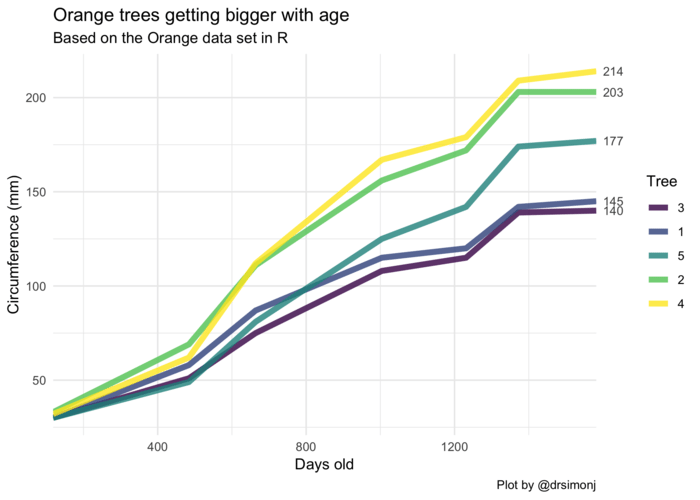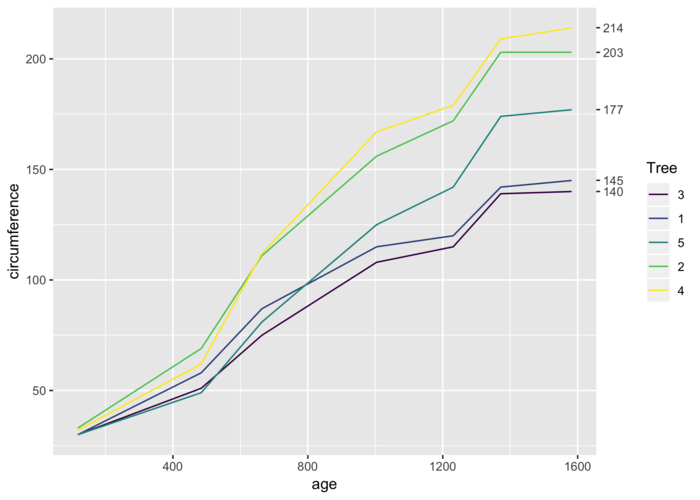# Label line ends in time series with ggplot2

September 25, 2018
By

Want to share your content on R-bloggers? click here if you have a blog, or here if you don't.

@drsimonj here with a quick share on making great use of the secondary y axis with ggplot2 – super helpful if you’re plotting groups of time series!

Here’s an example of what I want to show you how to create (pay attention to the numbers of the right):## Setup

To setup we’ll need the tidyverse package and the `Orange` data set that comes with R. This tracks the circumference growth of five orange trees over time.

``````library(tidyverse)

d <- Orange

#> Grouped Data: circumference ~ age | Tree
#>   Tree  age circumference
#> 1    1  118            30
#> 2    1  484            58
#> 3    1  664            87
#> 4    1 1004           115
#> 5    1 1231           120
#> 6    1 1372           142
``````

## Template code

To create the basic case where the numbers appear at the end of your time series lines, your code might look something like this:

``````# You have a data set with:
# - GROUP colum
# - X colum (say time)
# - Y column (the values of interest)
DATA_SET

# Create a vector of the last (furthest right) y-axis values for each group
DATA_SET_ENDS <- DATA_SET %>%
group_by(GROUP) %>%
top_n(1, X) %>%
pull(Y)

# Create plot with `sec.axis`
ggplot(DATA_SET, aes(X, Y, color = GROUP)) +
geom_line() +
scale_x_continuous(expand = c(0, 0)) +
scale_y_continuous(sec.axis = sec_axis(~ ., breaks = DATA_SET_ENDS))
``````

## Let’s see it!

Let’s break it down a bit. We already have our data set where the group colum is `Tree`, the X value is `age`, and the Y value is `circumference`.

So first get a vector of the last (furthest right) values for each group:

``````d_ends <- d %>%
group_by(Tree) %>%
top_n(1, age) %>%
pull(circumference)

d_ends
#>  145 203 140 214 177
``````

Next, let’s set up the basic plot without the numbers to see how each layer adds up.

``````ggplot(d, aes(age, circumference, color = Tree)) +
geom_line()
``````Now we can use `scale_y_*`, with the argument `sec.axis` to create a second axis on the right, with numbers to be displayed at `breaks`, defined by our vector of line ends:

``````ggplot(d, aes(age, circumference, color = Tree)) +
geom_line() +
scale_y_continuous(sec.axis = sec_axis(~ ., breaks = d_ends))
``````This is a great start, The only major addition I suggest is expanding the margins of the x-axis so the gap disappears. You do this with `scale_x_*` and the `expand` argument:

``````ggplot(d, aes(age, circumference, color = Tree)) +
geom_line() +
scale_y_continuous(sec.axis = sec_axis(~ ., breaks = d_ends)) +
scale_x_continuous(expand = c(0, 0))
``````## Polishing it up

Like it? Here’s the code to recreate the first polished plot:

``````library(tidyverse)

d <- Orange %>%
as_tibble()

d_ends <- d %>%
group_by(Tree) %>%
top_n(1, age) %>%
pull(circumference)

d %>%
ggplot(aes(age, circumference, color = Tree)) +
geom_line(size = 2, alpha = .8) +
theme_minimal() +
scale_x_continuous(expand = c(0, 0)) +
scale_y_continuous(sec.axis = sec_axis(~ ., breaks = d_ends)) +
ggtitle("Orange trees getting bigger with age",
subtitle = "Based on the Orange data set in R") +
labs(x = "Days old", y = "Circumference (mm)", caption = "Plot by @drsimonj")
``````## Sign off

Thanks for reading and I hope this was useful for you.

For updates of recent blog posts, follow @drsimonj on Twitter, or email me at [email protected] to get in touch.

If you’d like the code that produced this blog, check out the blogR GitHub repository.

R-bloggers.com offers daily e-mail updates about R news and tutorials about learning R and many other topics. Click here if you're looking to post or find an R/data-science job.
Want to share your content on R-bloggers? click here if you have a blog, or here if you don't.